# Some identities of generalized Tribonacci and Jacobsthal polynomials

Notes on Number Theory and Discrete Mathematics
Print ISSN 1310–5132, Online ISSN 2367–8275
Volume 27, 2021, Number 2, Pages 137—147
DOI: 10.7546/nntdm.2021.27.2.137-147

## Details

### Authors and affiliations

Abdeldjabar HamdiFaculty of Mathematics, University of Youcef Benkhedda Algiers 01
02 Rue Didouche Mourad, 16 000 Algeria

Salim Badidja ​Faculty of Mathematics, University of Kasdi Merbah
Ouargla Route de Ghardaia, BP. 511, 30 000 Algeria

### Abstract

In this study, we denote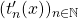the generalized Tribonacci polynomials, which are defined by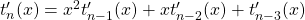,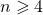, with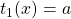,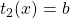,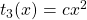and we drive an explicit formula ofin terms of their coefficients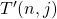, Also, we establish some properties of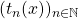. Similarly, we study the Jacobsthal polynomials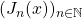, where,, with,and describe some properties.

### Keywords

• Tribonacci polynomials
• Generalized Tribonacci polynomials
• Jacobsthal polynomials

• 11B39
• 11B83

### References

1. Badidja, S., & Boudaoud, A. (2017). A unique representation of positive integers as a sum of distinct Tribonacci numbers. Journal of Mathematics and Statistics, 13(1), 57–61.
2. Badidja, S. (2018). Dèecomposition d’entiers illimitès et des termes d’ordre illimitès des suites recurrences linèaires. PhD thesis, University of Mohamed Boudiaf, Msila, Algeria.
3. Chelgham, M., & Boussayoud, A. (2020). Construction of symmetric functions of
generalized Tribonacci numbers. Journal of Science and Arts, 1(50), 65–74.
4. Gupta, Y. K., Badshah, V. H., Singh, M., & Sisodiya, K. (2016). Some identities of
Tribonacci polynomials. Turkish Journal of Analysis and Number Theory, 4(1), 20–22.
5. Koshy, T. (2001). Fibonacci and Lucas Numbers with Applications, Wiley-Interscience Publication.
6. Panwar, Y. K., Singh B., & Gupta V. K. (2013). Generalized Fibonacci polynomials. Turkish Journal of Analysis and Number Theory, 1(1), 43–47.
7. Rybołowicz, B., & Tereszkiewicz, A. (2018). Generalized Tricobsthal and generalized Tribonacci polynomials. Applied Mathematics and Computation, 325, 297–308.
8. Soykan, Y., & Okumus, I. (2019). On a generalized Tribonacci sequence. Journal of Progressive Research in Mathematics, 14(3), 1–9.

## Cite this paper

Hamdi, A., & Badidja, S. (2021). Some identities of generalized Tribonacci and Jacobsthal polynomials. Notes on Number Theory and Discrete Mathematics, 27(2), 137-147, doi: 10.7546/nntdm.2021.27.2.137-147.